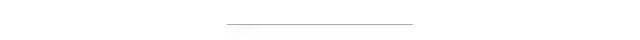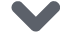# 两行代码统计模型参数量与FLOPs，这个PyTorch小工具值得一试• PyTorch-OpCounter GitHub 地址：https://github.com/Lyken17/pytorch-OpCounter

OpCouter

PyTorch-OpCounter 的安装和使用都非常简单，并且还能定制化统计规则，因此那些特殊的运算也能自定义地统计进去。

from torchvision.models import resnet50from thop import profilemodel = resnet50()input = torch.randn(1, 3, 224, 224)flops, params = profile(model, inputs=(input, ))flops: 2914598912.0parameters: 7978856.0

OpCouter 是怎么算的

def count_conv2d(m, x, y):    x = x    cin = m.in_channels    cout = m.out_channels    kh, kw = m.kernel_size    batch_size = x.size()    out_h = y.size(2)    out_w = y.size(3)    # ops per output element    # kernel_mul = kh * kw * cin    # kernel_add = kh * kw * cin - 1    kernel_ops = multiply_adds * kh * kw    bias_ops = 1 if m.bias is not None else 0    ops_per_element = kernel_ops + bias_ops    # total ops    # num_out_elements = y.numel()    output_elements = batch_size * out_w * out_h * cout    total_ops = output_elements * ops_per_element * cin // m.groups    m.total_ops = torch.Tensor([int(total_ops)])

class YourModule(nn.Module):    # your definitiondef count_your_model(model, x, y):    # your rule hereinput = torch.randn(1, 3, 224, 224)flops, params = profile(model, inputs=(input, ),                        custom_ops={YourModule: count_your_model})点击下方 |  | 了解更多